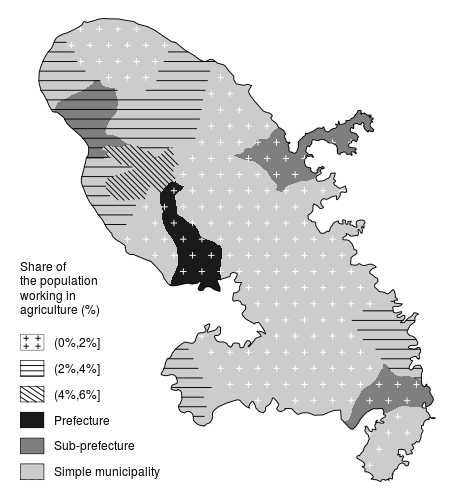# Beautiful Maps with R (III): Patterns and hatched maps

2019-12-12

On this post I would introduce a couple of functions that may be useful for improving your maps. The goal is to produce different filling patterns that could be added over your shapefiles.

At this point, I would like to suggest you (if you haven’t done it yet) to install the `cartography`package (vignette), as it presents a good bunch of interesting features, and fully compatible with the `sf` environment. In fact, I took some pieces of the base code in order to develop my own `legendPattern` function.

### Required R packages

``````library(sf)
library(dplyr)
library(rnaturalearth)``````

### Grid approach

I already worked with the `st_make_grid` on a previous post, and this time I have applied the same approach. Some examples here on how the grid can be used to create patterns:

#### Dots

``````DE <- ne_countries(50,
type = "countries",
country = "Germany",
returnclass = "sf"
) %>%
st_transform(3035)

grid <- st_make_grid(DE,
what = "corners",
square = F
)

# To avoid dots close to the edge
negbuff <- st_buffer(DE, dist = -15 * 1000)
grid2 <- grid[st_contains(negbuff, grid, sparse = F)]
par(mar = c(0, 0, 0, 0))
plot(st_geometry(DE))
plot(st_geometry(grid2), col = "red", add = T)``````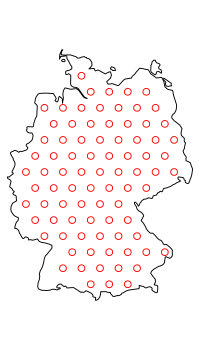#### Grid

``````# Grid
grid <- st_make_grid(DE,
what = "polygons",
square = T
) %>%
st_cast("LINESTRING") %>%
st_intersection(DE)

# Clean and keep lines only
grid2 <- grid[st_geometry_type(grid) %in% c("LINESTRING", "MULTILINESTRING")]

par(mar = c(0, 0, 0, 0))
plot(st_geometry(DE))
plot(st_geometry(grid2), col = "red", add = T)``````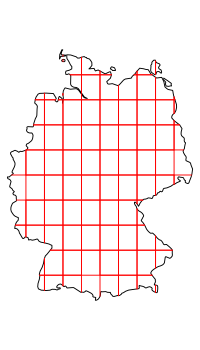Easy, right? Let’s move to the next level!

#### Horizontal line

``````# Horizontal
grid <- st_make_grid(DE,
what = "polygons",
square = T
)

par(mar = c(0, 0, 0, 0))
plot(grid)
plot(grid, add = T, col = "blue")
plot(
st_point(st_coordinates(grid)[1, 1:2]),
col = "red",
pch = 16
)

plot(
st_point(st_coordinates(grid)[2, 1:2]),
col = "orange",
pch = 16
)
plot(
st_point(st_coordinates(grid)[3, 1:2]),
col = "pink",
pch = 16
)
plot(
st_point(st_coordinates(grid)[4, 1:2]),
col = "black",
pch = 16
)``````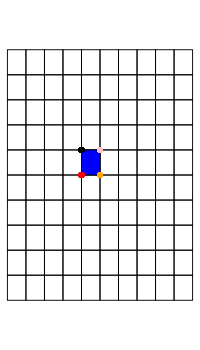As it can be seen, we can extract specific points of each grid. Once that I identified them it is just a matter of connecting points using the `st_linestring` function.

``````# Select horizontal only
grid_int <- lapply(
1:length(grid),
function(j)
st_linestring(st_coordinates(grid[j])[c(1, 2), 1:2])
) %>%
st_sfc(crs = st_crs(DE)) %>%
st_intersection(DE)

# Clean and keep lines only
grid2 <- grid_int[st_geometry_type(grid_int) %in% c("LINESTRING", "MULTILINESTRING")]

par(mar = c(0, 0, 0, 0))
plot(st_geometry(DE))
plot(st_geometry(grid2), col = "red", add = T)``````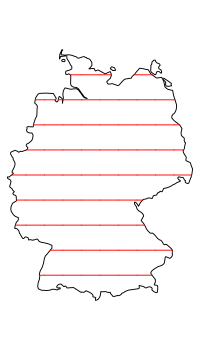### The `patternLayer` function

I put all that together on a function named `patternLayer` (link). The main inputs are:

• `x`: `sf` object. It must be a `POLYGON` or a `MULTIPOLYGON`.
• `pattern`: fillings available are `c("dot", "text", "diamond", "grid", "hexagon", "horizontal", "vertical", "zigzag", "left2right", "right2left", "circle")`.
• `density` of the grid. By default the function uses a grid with a minimum of 10 cells on the shortest dimension of the bounding box. Additionally it is possible to pass a `cellsize` value that would feed the `st_make_grid` underlying function.
• `txt` for the `text` pattern, that should be a character.
• Different graphical parameters can be also passed, as `add`, `cex`, `lty`, `pch`, etc. Basically three modes are allowed: points, lines and texts.

Let’s see how it works.

``````# Load function
source("../assets/functions/patternfun.R")

par(
mfrow = c(3, 4),
mar = c(1, 1, 1, 1),
cex = 0.5
)
patternLayer(DE, "dot")
title("dot")
patternLayer(DE, "text", txt = "Y")
title("text")
patternLayer(DE, "diamond", density = 0.5)
title("diamond")
patternLayer(DE, "grid", lwd = 1.5)
title("grid")
patternLayer(DE, "hexagon", col = "blue")
title("hexagon")
patternLayer(DE, "horizontal", lty = 5)
title("horizontal")
patternLayer(DE, "vertical")
title("vertical")
patternLayer(DE, "left2right")
title("left2right")
patternLayer(DE, "right2left")
title("right2left")
patternLayer(DE, "zigzag")
title("zigzag")
patternLayer(DE, "circle")
title("circle")``````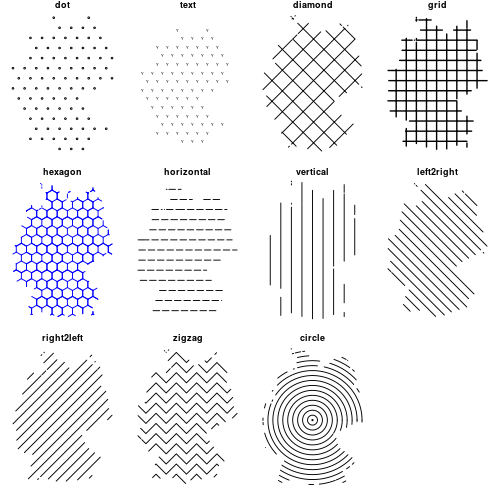Let’s play a little bit more with some of the additional features of the function:

``````par(mar = c(1, 1, 1, 1), mfrow = c(2, 3))
plot(st_geometry(DE))
patternLayer(
DE,
"dot",
pch = 10,
density = 0.5,
cex = 2,
col = "darkblue",
)
plot(st_geometry(DE))
patternLayer(
DE,
"dot",
pch = 21,
col = "red",
bg = "green",
cex = 1.25,
)
plot(st_geometry(DE), col = "grey")
patternLayer(
DE,
"text",
txt = "DE",
density = 1.1,
col = "white",
)
plot(st_geometry(DE), col = "blue")
patternLayer(
DE,
"horizontal",
lty = 3,
cellsize = 150 * 1000,
)
patternLayer(DE, "zigzag", lwd = 2, col = "red")
plot(st_geometry(DE), border = "orange", lwd = 2)
patternLayer(DE,
"left2right",
density = 2,
col = "orange",
)``````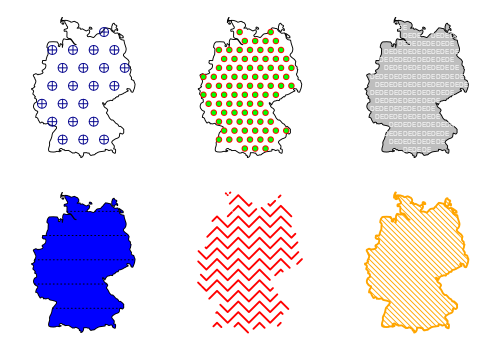### Adding legends: the `legendPattern` function

As a complementary function, I created also the `legendPattern` function, heavily based on the `legends.R` script developed by @riatelab for the `cartography` package (source code).

Main parameters are:

• `pos`, `title.txt`, `title.cex`, `values.cex`,`categ`, `cex` and `frame`: See `?cartography::legendTypo`.
• `patterns`: vector of patterns to be created for each element on `categ`.
• `ptrn.bg`: Background of the legend box for each `categ`.
• `ptrn.text`: Text to be used for each `categ="text"`, as a single value or a vector.
• `dot.cex`: `cex` of each `categ="dot"`, as a single value or a vector.
• `text.cex`: text size of each `categ="text"`, as a single value or a vector.
• As in the case of the `patternLayer`function, different graphical parameters can be passed (`lty`, `lwd`, `pch`, `bg` on points).

Note that is also possible to create solid legends, by setting `col` and `ptrn.bg` to the same color. Parameters would respect the order of the `categ` variable.

``````par(mar = c(0, 0, 0, 0), mfrow = c(1, 1))
plot(st_geometry(DE)) # Null geometry
legendPattern(
title.txt = "Example 1",
categ = c("a", "b"),
patterns = "dot",
pch = c(16, 23),
frame = T
)
legendPattern(
pos = "left",
title.txt = "Example 2",
categ = c("c", "d", "other text"),
patterns = c("text", "zigzag"),
ptrn.text = c("s", "pp"),
ptrn.bg = "grey80",
col = c("red", "blue")
)

legendPattern(
pos = "topright",
title.txt = "Example 3",
categ = c("e", "f", "solid"),
patterns = c("circle", "left2right"),
ptrn.bg = c("orange", "yellow", "green"),
col = c("white", "white", "green"),
lty = c(2, 4),
lwd = c(1, 3)
)

legendPattern(
pos = "bottomright",
title.txt = "Example 4",
values.cex = 1.2,
categ = c("h", "i", "j", "k"),
patterns = c("grid", "diamond", "horizontal", "dot"),
cex = 2,
pch = 22,
col = "white",
ptrn.bg = "black",
bg = "pink"
)``````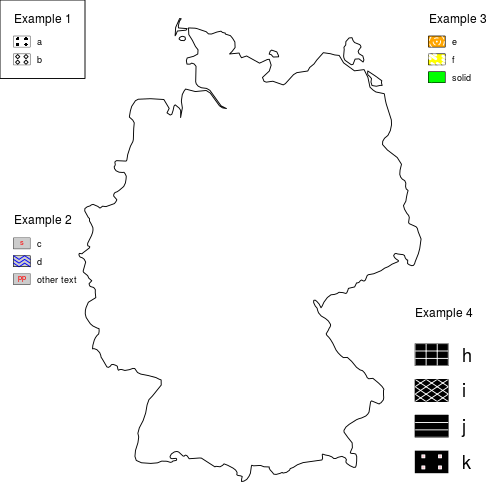I hope that you find this functions useful. Enjoy and nice mapping!